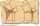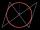# The right triangle altitude theorem - math problems

The altitude to the hypotenuse is the geometric mean of the two segments of the hypotenuse. Each leg of the right triangle is the mean proportional of the hypotenuse and the segment of the hypotenuse that is adjacent to the leg.

$h=\sqrt{{c}_{1}{c}_{2}}$

Also known as a geometric mean theorem. The geometric mean theorem is a special case of the chord theorem.

#### Number of problems found: 48

• Area of RTThe right triangle has orthogonal projections of legs to the hypotenuse lengths 15 cm and 9 cm. Determine the area of ​​this triangle.
• Euclid2In the right triangle ABC with a right angle at C is given side a=29 and height v=17. Calculate the perimeter of the triangle.
• Euclid3Calculate height and sides of the right triangle, if one leg is a = 81 cm and section of hypotenuse adjacent to the second leg cb = 39 cm.
• Euclid1Right triangle has hypotenuse c = 27 cm. How large sections cuts height hc=3 cm on the hypotenuse c?
• TangentsTo circle with a radius of 41 cm from the point R guided two tangents. The distance of both points of contact is 16 cm. Calculate the distance from point R and circle centre.
• RT - hypotenuse and altitudeRight triangle BTG has hypotenuse g=117 m and altitude to g is 54 m. How long are hypotenuse segments?
• Triangle ABCRight triangle ABC with right angle at the C, |BC|=18, |AB|=33. Calculate the height of the triangle hAB to the side AB.
• Circle in rhombusIn the rhombus is an inscribed circle. Contact points of touch divide the sides to parts of length 19 cm and 6 cm. Calculate the circle area.

We apologize, but in this category are not a lot of examples.
Do you have an exciting math question or word problem that you can't solve? Ask a question or post a math problem, and we can try to solve it.

We will send a solution to your e-mail address. Solved examples are also published here. Please enter the e-mail correctly and check whether you don't have a full mailbox.